Search

Systems of Linear Equations:
Solving by Gaussian Elimination
(page 6 of 7)

Sections: Definitions, Solving by graphing, Substitition, Elimination/addition, Gaussian elimination.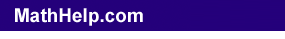Solving three-variable, three-equation linear systems is more difficult, at least initially, than solving the two-variable systems, because the computations involved are more messy. You will need to be very neat in your working, and you should plan to use lots of scratch paper. The method for solving these systems is an extension of the two-variable solving-by-addition method, so make sure you know this method well and can use it consistently correctly.

Though the method of solution is based on addition/elimination, trying to do actual addition tends to get very messy, so there is a systematized method for solving the three-or-more-variables systems. This method is called "Gaussian elimination" (with the equations ending up in what is called "row-echelon form").

Let's start simple, and work our way up to messier examples.

• Solve the following system of equations.
• 5x + 4y     z = 0
10
y  3z = 11

z = 3

It's fairly easy to see how to proceed in this case. I'll just back-substitute the z-value from the third equation into the second equation, solve the result for y, and then plug z and y into the first equation and solve the result for x.

10y  3(3) = 11
10y  9 = 11
10y = 20
y = 2

5x + 4(2)  (3) = 0
5x + 8  3 = 0
5x + 5 = 0
5x = 5
x = 1

Then the solution is (x, y, z) = (1, 2, 3).

The reason this system was easy to solve is that the system was "triangular"; this refers to the equations having the form of a triangle, because of the lower equations containing only the later variables.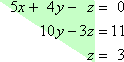The point is that, in this format, the system is simple to solve. And Gaussian elimination is the method we'll use to convert systems to this upper triangular form, using the row operations we learned when we did the addition method.

• Solve the following system of equations using Gaussian elimination.
• 3x + 2y  6z = 6
5
x + 7y  5z = 6

x + 4y  2z = 8

No equation is solved for a variable, so I'll have to do the multiplication-and-addition thing to simplify this system. In order to keep track of my work, I'll write down each step as I go. But I'll do my computations on scratch paper. Here is how I did it:

The first thing to do is to get rid of the leading x-terms in two of the rows. For now, I'll just look at which rows will be easy to clear out; I can switch rows later to get the system into "upper triangular" form. There is no rule that says I have to use the x-term from the first row, and, in this case, I think it will be simpler to use the x-term from the third row, since its coefficient is simply "1". So I'll multiply the third row by 3, and add it to the first row. I do the computations on scratch paper: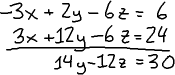...and then I write down the results: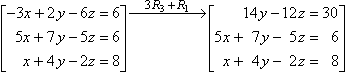(When we were solving two-variable systems, we could multiply a row, rewriting the system off to the side, and then add down. There is no space for this in a three-variable system, which is why we need the scratch paper.)

Warning: Since I didn't actually do anything to the third row, I copied it down, unchanged, into the new matrix of equations. I used the third row, but I didn't actually change it. Don't confuse "using" with "changing".

To get smaller numbers for coefficients, I'll multiply the first row by one-half: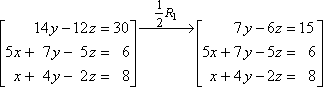Now I'll multiply the third row by 5 and add this to the second row. I do my work on scratch paper: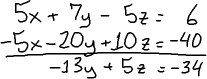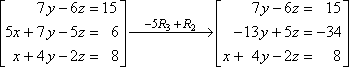I didn't do anything with the first row, so I copied it down unchanged. I worked with the third row, but I only worked on the second row, so the second row is updated and the third row is copied over unchanged.

Okay, now the x-column is cleared out except for the leading term in the third row. So next I have to work on the y-column.

Warning: Since the third equation has an x-term, I cannot use it on either of the other two equations any more (or I'll undo my progress). I can work on the equation, but not with it.

If I add twice the first row to the second row, this will give me a leading 1 in the second row. I won't have gotten rid of the leading y-term in the second row, but I will have converted it (without getting involved in fractions) to a form that is simpler to deal with. (You should keep an eye out for this sort of simplification.) First I do the scratch work: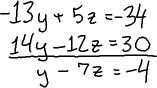...and then I write down the results: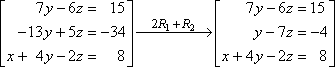Now I can use the second row to clear out the y-term in the first row. I'll multiply the second row by 7 and add. First I do the scratch work: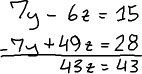...and then I write down the results: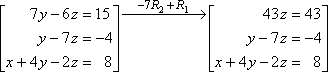I can tell what z is now, but, just to be thorough, I'll divide the first row by 43. Then I'll rearrange the rows to put them in upper-triangular form: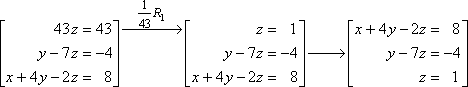Now I can start the process of back-solving:

y  7(1) = 4
y  7 = 4
y = 3

x + 4(3)  2(1) = 8
x + 12  2 = 8
x + 10 = 8
x = 2

Then the solution is (x, y, z) = (2, 3, 1).

Note: There is nothing sacred about the steps I used in solving the above system; there was nothing special about how I solved this system. You could work in a different order or simplify different rows, and still come up with the correct answer. These systems are sufficiently complicated that there is unlikely to be one right way of computing the answer. So don't stress over "how did she know to do that next?", because there is no rule. I just did whatever struck my fancy; I did whatever seemed simplest or whatever came to mind first. Don't worry if you would have used completely different steps. As long as each step along the way is correct, you'll come up with the same answer.

Content Continues Below

In the above example, I could have gone further in my computations and been more thorough-going in my row operations, clearing out all the y-terms other than that in the second row and all the z-terms other than that in the first row. This is what the process would then have looked like: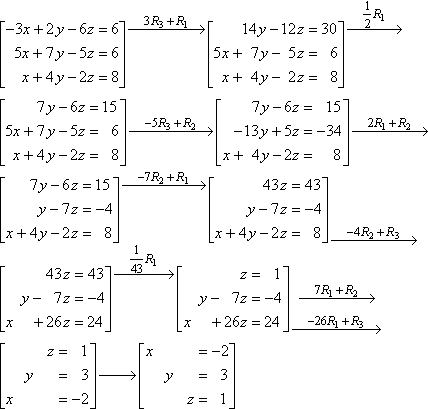This way, I can just read off the values of x, y, and z, and I don't have to bother with the back-substitution. This more-complete method of solving is called "Gauss-Jordan elimination" (with the equations ending up in what is called "reduced-row-echelon form"). Many texts only go as far as Gaussian elimination, but I've always found it easier to continue on and do Gauss-Jordan.

Note that I did two row operations at once in that last step before switching the rows. As long as I'm not working with and working on the same row in the same step, this is okay. In this case, I was working with the first row and working on the second and third rows.

<< Previous  Top  |  1 | 2 | 3 | 4 | 5 | 6 | 7  |  Return to Index  Next >>

 Cite this article as: Stapel, Elizabeth. "Systems of Linear EquationsL Solving By Gaussian Elimination." Purplemath     Available from https://www.purplemath.com/modules/systlin6.htm.     Accessed [Date] [Month] 2016

Purplemath:

Study Skills Survey